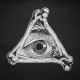# Introduction to Probability and StatisticsLast Update November 6, 2020
5.0 /5
(1)

This course will begin with an overview of data types and descriptive Statistics. There will be extensive coverage of probability topics along with an introduction to discrete and continuous probability distributions. The course ends with a discussion of the central limit theorem and coverage of estimation using confidence intervals and hypothesis testing. This course is equivalent to most college level Statistics I courses.

#### Learning Objectives

Learn the probability and basic techniques of data analysis and inference.
Gain a deeper understanding of the underlying concepts.

#### Requirements

• Basic Arithmetic and Algebra Skills

#### Target Audience

• Students that are taking or would like to take an introductory course in Statistics in college
• AP courses in high school will find this course useful.

8h 29m

#### Probability

4.94/5
202 Courses
54 Reviews
232 Students
See more

\$45.00

Level
Beginner
Duration 8.5 hours
Subject

Related Courses

\$45.00

\$45.00

##### Introduction to Probability and Statistics

\$45.00

Select the fields to be shown. Others will be hidden. Drag and drop to rearrange the order.
• Image
• SKU
• Rating
• Price
• Stock
• Availability